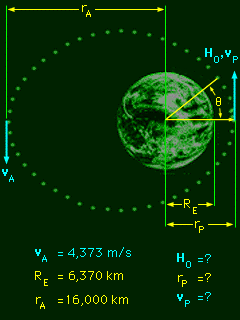Ch 4. Particle Momentum and Impulse Multimedia Engineering Dynamics Impulse & Momentum Consv. Linear Momentum Impact AngularMomentum MassFlow
 Chapter - Particle - 1. General Motion 2. Force & Accel. 3. Energy 4. Momentum - Rigid Body - 5. General Motion 6. Force & Accel. 7. Energy 8. Momentum 9. 3-D Motion 10. Vibrations Appendix Basic Math Units Basic Equations Sections Search eBooks Dynamics Fluids Math Mechanics Statics Thermodynamics Author(s): Kurt Gramoll ©Kurt GramollDYNAMICS - CASE STUDY IntroductionProblem Diagram After docking with the Soyuz, the Apollo must re-enter the earth's atmosphere from perigee (the closest point of the orbital path to the earth). If the Apollo is traveling too fast, it will burn up during re-entry. What is known: The mass of the Apollo is mA = 18.0 Mg. At apogee (the furthest point of the orbital path from the earth), the speed of the Apollo is vA = 4,373 m/s. At apogee, the Apollo's distance from the center of the earth is rA = 16,000 km. The radius of the earth is RE = 6,370 km. Question At perigee, what is the Apollo's angular momentum, distance from the center of the earth, and speed? Approach Use the equations of angular momentum to determine the angular momentum at apogee, then at perigee. Use equations involving the distance from the center of the earth and the transverse velocity (derived from angular momentum) to find the velocity at perigee.

Practice Homework and Test problems now available in the 'Eng Dynamics' mobile app
Includes over 400 problems with complete detailed solutions.
Available now at the Google Play Store and Apple App Store.